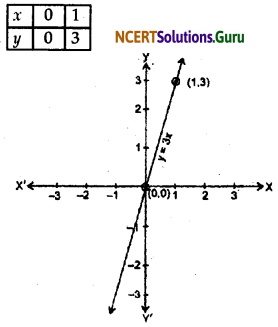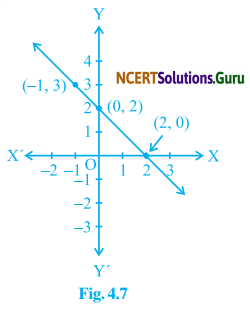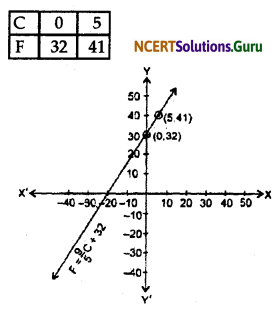# NCERT Solutions for Class 9 Maths Chapter 4 Linear Equations in Two Variables Ex 4.3

These NCERT Solutions for Class 9 Maths Chapter 4 Linear Equations in Two Variables Ex 4.3 Questions and Answers are prepared by our highly skilled subject experts.

## NCERT Solutions for Class 9 Maths Chapter 4 Linear Equations in Two Variables Exercise 4.3

Question 1.
Draw the graph of each of the following linear equations in two variables:
(i) x + y = 4
(ii) x – y = 2
(iii) y = 3x
(iv) 3 = 2x + y
Solution:
(i) We have given the equation
x + y = 4
To draw the graph, we need at least two solutions to the equation.
Put x = 0 then y = 4 and if x = 4 then y = 0 are solutions of the given equation.
So we use the following table to draw the graph:(ii) We have given the equation x – y = 2
To draw the graph, we need at least two solutions to the equation.
Put x = 0 then y = -2 and if x = 2 then y = 0 are solutions of the given equation.
So we use the following table to draw the graph:(iii) We have given the equation y = 3x
To draw the graph, we need at least two solutions to the equation.
Put x = 0 the y = 0, and if x = 1 then y = 3 are the solutions of the given equation.
So we use the following table to draw the graph:(iv) We have given the equation 3 = 2x + y
To draw the graph, we need at least two solutions to the equation.
Put x = 0 then y = 3 and if x = 1 then y = 1 are the solutions of the given equation.
So we use the table to draw the graph:Question 2.
Give the equations of two lines passing through (2, 14). How many more such lines are there, and why?
Solution:
Here (2, 14) is the solution of a linear equation which is satisfied by the co-ordinate of the point (2,14).
For eg. 2x + 10 = y, 3x + 8 = y, 4x + 6 = y and so on.
It is because we know that from a given point there are infinitely many straight lines that pass.Question 3.
If the point (3, 4) lies on the graph of the equation 3y = ax + 7, find the value of a?
Solution:
We have given that (3, 4) lies on the graph of the equation.
Therefore we put x = 3 and y = 4 in this equation.
3 × 4 = a × 3 + 7
or, 12 = 3a + 7
or, 12 – 7 = 3a
or, a = $$\frac {5}{3}$$
Therefore, the value of a = $$\frac {5}{3}$$

Question 4.
The taxi fare in a city is as follows:
For the first kilometer, the fare is Rs. 8 and for the subsequent distance, it is Rs. 5 per km. Taking the distance covered as x km and total fare is Rs. y, write a linear equation for this information and draw its graph.
Solution:
We have given that the total distance covered is x km and the total fare is Rs. y.
But, we have given that the rate of the first km is Rs. 8.
Therefore the fare of the remaining distance (x – 1) km is Rs. 5 per km.
Therefore, according to question, we can say
(x – 1) × 5 + 8 = y
or, 5x – 5 + 8 = y
or, 5x + 3 = y
Therefore, 5x + 3 = y is required equation for this information.
We use the following table to represent the graph.Question 5.
From the choices given below, choose the equation whose graphs are given in Fig. 4.6 and Fig. 4.7.
(a) For Fig. 4.6
(i) y = x
(ii) x + y = 0
(iii) y = 2x
(iv) 2 + 3y = 7x(b) For Fig. 4.7
(i) y = x + 2
(ii) y = x – 2
(iii) y = -x + 2
(iv) x + 2y = 6Solution:
(a) In Fig. 4.6 the points on the line are (-1, 1), (0, 0) and (1, -1). By inspection x + y = 0 is the equation corresponding to this graph. We find that the coordinate in each case is equal but an opposite sign of the x coordinate.

(b) In Fig. 4.7 the points on the line are (0, 2) and (2, 0). By inspection y = -x + 2 is the equation corresponding to this graph. We find that when we put x = 0 then the value of y = 2 and when we put x = 2 then the value of y = 0.Question 6.
If the work done by a body on the application of a constant force is directly proportional to the distance travelled by the body. Express this in the form of an equation in two variables and draw the graph of the same by taking the constant force as 5 units. Also real from the graph the work done when the distance travelled by the body is
(i) 2 units
(ii) 0 units
Solution:
Let us assume,
The work done by a constant force is y-units and the distance travelled by the body is x-units. Therefore, According to the question.
Force y is directly proportional to distance travelled x
y ∝ x
y = kx (where k is constant)
Again according to the question.
We have given that constant force (k) = 5 units.
The required two-variable equation is y = 5x
(i) According to the graph,
When the distance travelled by the body is 2 units, then the work done is 10 units.
(ii) According to the graph,
When the distance travelled by the body is 0 units, the work done is 0 Units.
To draw the graph of y = 5x we use the following table.Question 7.
Yamini and Fatima, two students of class IX of a school, together contributed ₹ 100 towards Prime Minister’s Relief Fund to help the earthquake victims. Write a linear equation which satisfies this data, (you may take their contributions as ₹ x and ₹ y). Draw the graph of the same.
Solution:
Let Yamini contributed towards Prime Minister Relief Fund is ₹ x and Fatima Contributed ₹ y.
Therefore, According to question,
x + y = 100
The linear equation which satisfies this data is x + y = 100.
To draw the graph we use the following table:Question 8.
In countries like the USA and Canada, the temperature is measured in Fahrenheit, whereas in countries like India it is measured in Celsius. Here is a linear equation that converts Fahrenheit to Celsius:
F = ($$\frac {9}{5}$$) C + 32
(i) Draw the graph of the linear equation above using Celsius for the x-axis and Fahrenheit for the y-axis.
(ii) If the temperature is 30°C, what is the temperature in Fahrenheit?
(iii) If the temperature is 95° F, what is the temperature in Celsius?
(iv) If the temperature is 0°C, what is the temperature in Fahrenheit and if the temperature is 0°F, what is the temperature in Celsius?
(v) Is there a temperature which is numerically the same in both Fahrenheit and Celsius? If yes find it.
Solution:
(i) We have given that the linear equation.
F = ($$\frac {9}{5}$$) C + 32
To draw the graph, we need at least two solutions to the equation.
Put C = 0 then F = 32 and if C = 5 then F = 41 are the solutions of the given equation.
So we use the following table to draw the graph.Taking C on the x-axis and F on the y-axis.

(ii) We have given that C = 30°
Then by the linear equation F = ($$\frac {9}{5}$$) C + 32
Put C = 30
F = $$\frac {9}{5}$$ × 30 + 32
F = 54 + 32
∴ F = 86
Therefore, If the temperature is 30°C then the temperature in Fahrenheit is 86°.

(iii) We have given that F = 95°
Then by the linear equation
F = ($$\frac {9}{5}$$) C + 32
Put F = 95°
or, 95 = $$\frac {9}{5}$$ C + 32
or, 95 – 32 = ($$\frac {9}{5}$$) C
63 × $$\frac {5}{9}$$ = C
∴ C = 35°
The temperature of 95°F is 35° Celsius.(iv) We have given that C = 0°
Then by the linear equation
F = ($$\frac {9}{5}$$) C + 32
Put C = 0°
F= ($$\frac {5}{9}$$) × 0 + 32
or, F = 0 + 32
F = 32
The temperature of 0°C is 32 Fahrenheit.
Again in case II,
We have given that F = 0°
Then by liner equation
F = ($$\frac {9}{5}$$) C + 32
put F = 0
0 = ($$\frac {9}{5}$$) C + 32
or, 0 – 32 = ($$\frac {9}{5}$$) C
or, -32 × $$\frac {5}{9}$$ = C
or, $$\frac {-160}{9}$$ = C
C = -17.77
or, C = 17.7
∴ The temperature of 0°F is -17.7 Celsius.(v) Yes, there is a temperature which is numerically the same in both Fahrenheit and Celsius.
This is -40°C
At -40°C the value of the Fahrenheit scale is also -40°F.

error: Content is protected !!